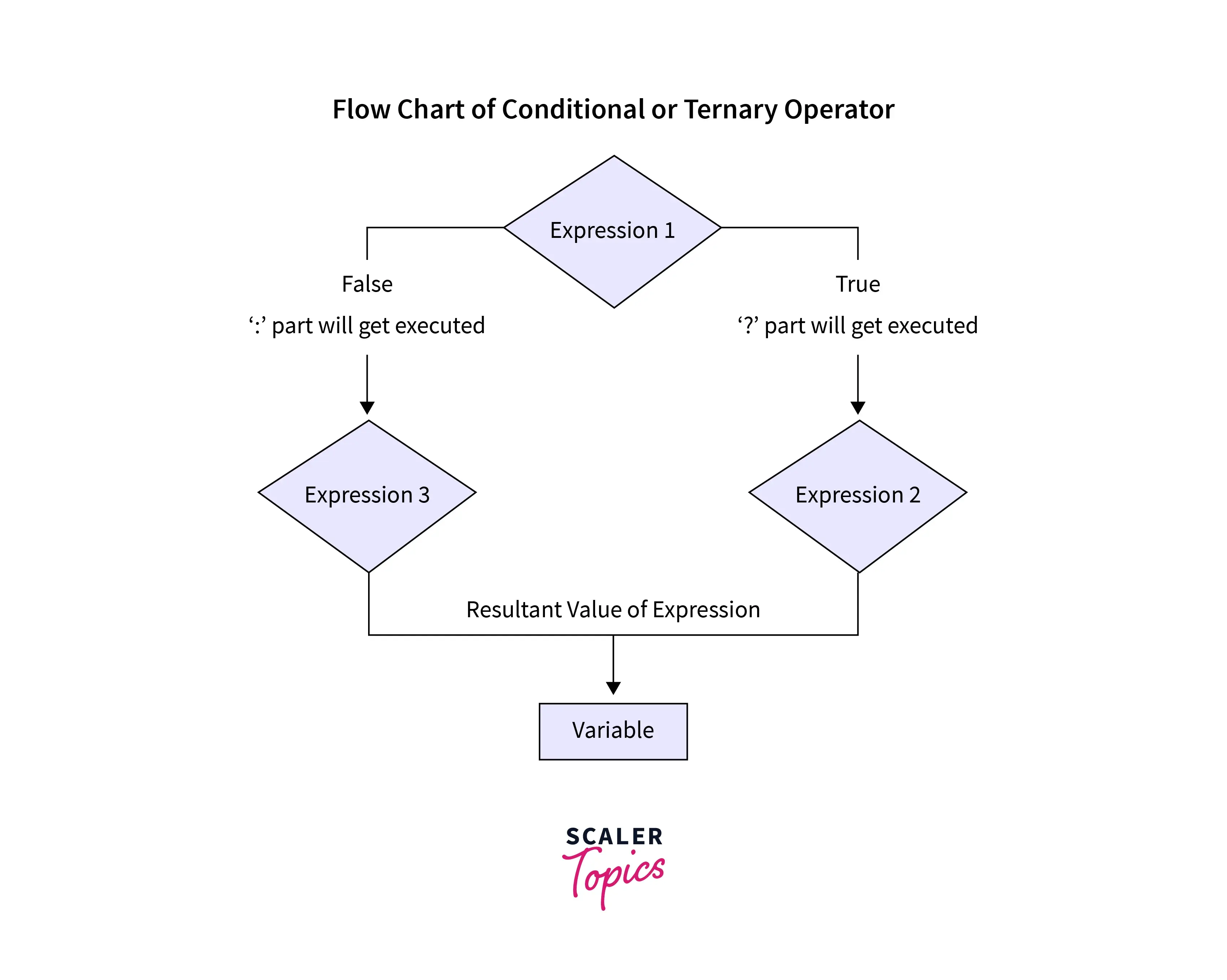# Ternary Operator in Java

Challenge Inside! : Find out where you stand! Try quiz, solve problems & win rewards!
Video TutorialTernary operator in Java is used as a replacement for the if-then-else statement. It simulates this behavior - If expression 1 is true, variable = expression2 else variable = expression3. It is the only operator in Java that takes three arguments.

## Ternary Operator

Operators in Java are symbols that instruct the compiler to perform a specific operation. Just like other operators like Arithmetic, Bitwise, Unary, etc, Ternary Operator adds up to the list of operators available in Java.

• If statement is too much code

We usually write conditional statements in our codes every now and then, as they play an important role in deciding some specific path of the workflow or often decide between multiple actions based on the condition.

Ternary operator allows us to translate multiple lines of code of an ‘if-else’ block into a single line. As a clean code developer, you might always question, if it’s a one liner if-else, is it readable? Yes, it’s readable and structured more than an if-else block. Let’s see how it really does that with its syntax.

## Syntax

Condition: It represents the condition that is written in an if statement.

Expression1: This expression will be stored in the Variable if the condition turns out to be true.

Expression2: This expression will be stored in the Variable if the condition turns out to be false.

Variable: It stores the value returned by either expression.

### Flowchart of Ternary Operation## Example

Output:

The above piece of code can be reduced drastically to just one line using the Ternary operator in Java:

Output:

## When to Use a Ternary Operator in Java?

• The Ternary operator in Java is a concise alternative to writing conditional statements, serving as a shorthand for if-else implementations.
• It's important to store the value in a variable when using the Ternary operator, as each expression within the operator returns a value.
• Storing the result in a variable becomes necessary when the conditional statement is intended to modify a specific variable or return a specific data type.

In such cases, the Ternary operator is the preferred choice. For example,

If-else statement:

The above code can be done in two lines using ternary operator as shown below:

Ternary operator:

## Nested Ternary Operator

The nested ternary operator is an extension of the ternary operator in Java, allowing for multiple levels of conditionals within a single expression. It provides a compact way to handle complex conditional logic.

Here's a short code example:

Output:

• In this example, the nested ternary operator is used to determine the value of the result variable based on the value of x.
• The outer condition (x > 0) checks if x is greater than zero.
• If true, it proceeds to the inner ternary operator (x < 10), which checks if x is less than ten. I
• f true, the result is "x is less than 10", otherwise it is "x is greater than or equal to 10". If the outer condition is false, the result is "x is less than or equal to 0".